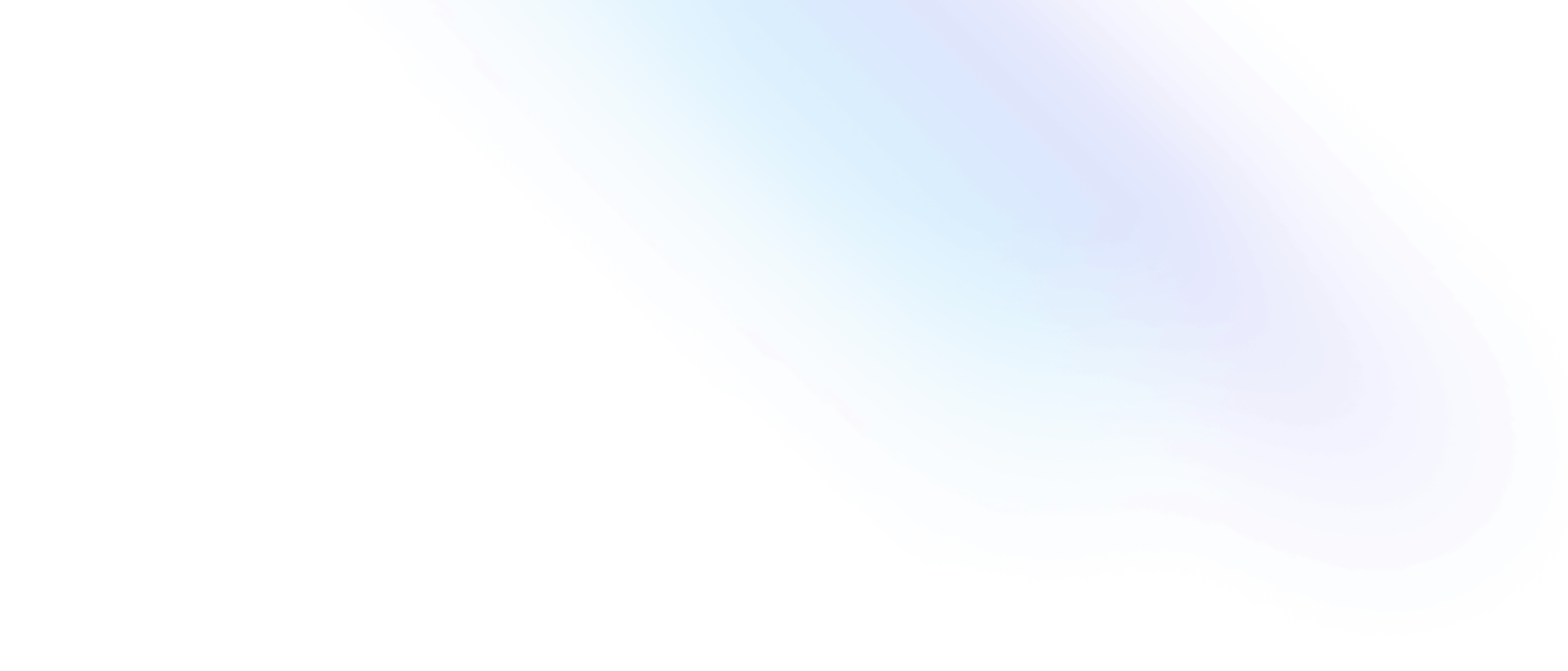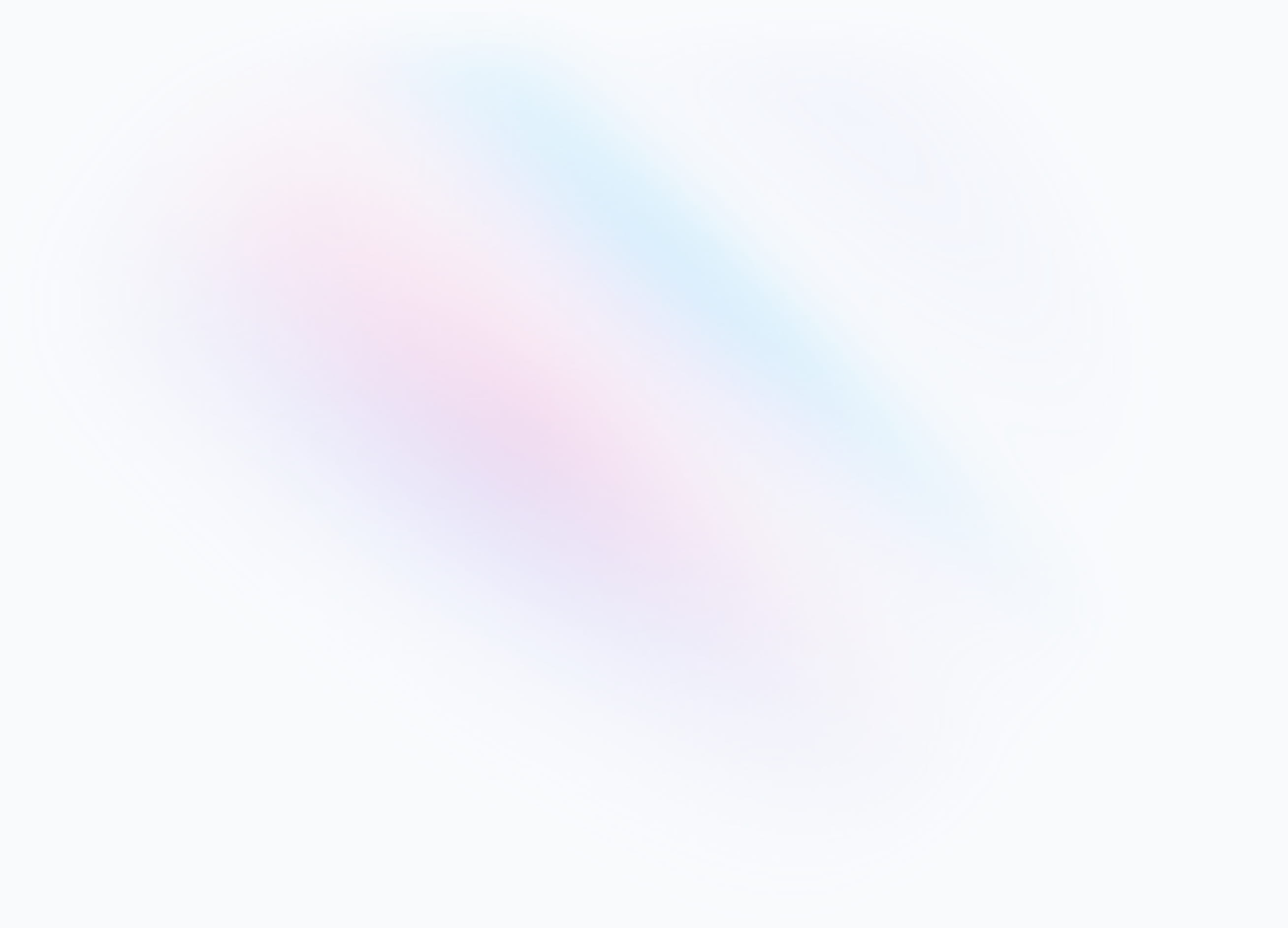# How to Crop image in Golang?self.__wrap_balancer=(t,e,n)=>{n=n||document.querySelector(`[data-br="\${t}"]`);let o=n.parentElement,r=E=>n.style.maxWidth=E+"px";n.style.maxWidth="";let i=o.clientWidth,s=o.clientHeight,c=i/2,u=i,d;if(i){for(;c+1<u;)d=~~((c+u)/2),r(d),o.clientHeight==s?u=d:c=d;r(u*e+i*(1-e))}};self.__wrap_balancer(":R2sm:",1)

To crop an image in Go, you can use the `image.SubImage` method in the image package to create a new image that is a sub-rectangle of the original image. This method takes one argument: reactangle coordinates of the new image. We can use `image.Rectagle` module to provide to coordinate of the new image we want to crop.

## Interface

If you see when we create an instance of image which is can be `png` or `jpg` we can not find `SubImage` method. But it is available on the image package, to solve this we can use some custom interface to cast the `SubImage` method.

``````type SubImager interface {
SubImage(r image.Rectangle) image.Image
}
``````

Now let's open the image and parse it to `jpg` or `png`. In this article we will use `png`. So, let's import the package we need.

``````import (
"image"
"image/png"
"os"
)
``````

Replace `"image/png"` with `"image/ppg"` if you are working with jpg image or you can also import both package if you are working with both image format.

To read files we can use `os` package like this.

``````func main() {
originalImageFile, err := os.Open("original.png")
if err != nil {
panic(err)
}
defer originalImageFile.Close()

originalImage, err := png.Decode(originalImageFile)
if err != nil {
panic(err)
}
...
}
``````

## Crop the Image

Here's an example image we will working with.So here's the code to crop the images.

``````bounds := originalImage.Bounds()
width := bounds.Dx()
// height := bounds.Dy() you can use this to work with the height of the images
cropSize := image.Rect(0, 0, width/2+100, width/2+100)
croppedImage := originalImage.(SubImager).SubImage(cropSize)
``````

It will be cropped to this.Explanation:

• `image.Rect`: Since we need to pass `image.Rectangle` to method `SubImage` we can use this module to generate rectangle coordinate for us.
• `image.Rect(0, 0, width/2+100, width/2+100)`: Is the width and height of the new cropped image size. In this case we are divide the current width image by half and add `100` more pixels. You can changes the value what ever you want.
• `cropSize.Add(image.Point{100, 80})`: This is the place of the left and top padding of image that you want to crop. In this case we add padding left to `100` pixels and padding top of `80` pixels.

## Save image

After cropping the image the next step is to save it. And here's how you can save the image to a file.

``````croppedImageFile, err := os.Create("cropped.png")
if err != nil {
panic(err)
}

defer croppedImageFile.Close()
if err := png.Encode(croppedImageFile, croppedImage); err != nil {
panic(err)
}
``````

You can change `png.Encode` to `jpg.Encode` if you work with jpg file.

Here is the complete code for this example.

``````package main

import (
"image"
"image/png"
"os"
)

type SubImager interface {
SubImage(r image.Rectangle) image.Image
}

func main() {
originalImageFile, err := os.Open("original.png")
if err != nil {
panic(err)
}
defer originalImageFile.Close()

originalImage, err := png.Decode(originalImageFile)
if err != nil {
panic(err)
}

bounds := originalImage.Bounds()
width := bounds.Dx()
cropSize := image.Rect(0, 0, width/2+100, width/2+100)
croppedImage := originalImage.(SubImager).SubImage(cropSize)

croppedImageFile, err := os.Create("cropped.png")
if err != nil {
panic(err)
}

defer croppedImageFile.Close()
if err := png.Encode(croppedImageFile, croppedImage); err != nil {
panic(err)
}
}
``````

Bonus: I have created this simple cli to crop image with go check it out here: https://github.com/ahmadrosid/crop

I hope this tutorial was helpful and if you have any questions, shoot me a DM on Twitter.

Built with Next.js, Tailwind and Vercel